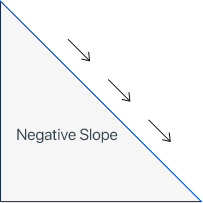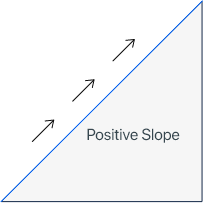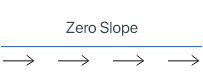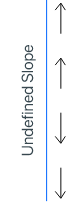# Types

## The negative slope of the line

It is a type of slope in which the value or the result of the slope will be negative. The negative slope means a person or an object moving from left to right falls or goes upward.Example

What is the slope of the line of (-14, -8) and (-11, -22)?

Solution

Step 1: Take the given points of the line.

x1 = -14, x = -11, y1 = -8, and y2 = -22

Step 2: Write the formula of slope of the line.

Slope of the line = m = Δy/Δx = (y2-y1)/(x2-x1)

Step 3: Put the values of x1, x2, y1, and y2 in the above formula to calculate the slope.

Slope of the line = m = (-22 – (-8))/(-11 – (-14))

Slope of the line = m = (-22 + 4)/(-11 + 14)

Slope of the line = m = (-18)/(3)

Slope of the line = m = -4.67

The slope of the line of the given two points is -4.67.

## The positive slope of the line

It is a type of slope in which the value or the result of the slope will be positive. The positive slope means a person or an object moving from left to right to climb up or go upward.Example

What is the slope of the line of (-4, -7) and (-11, -12)?

Solution

Step 1: Take the given points of the line.

x1 = -4, x = -11, y1 = -7, and y2 = -12

Step 2: Write the formula of slope of the line.

Slope of the line = m = Δy/Δx = (y2-y1)/(x2-x1)

Step 3: Put the values of x1, x2, y1, and y2 in the above formula to calculate the slope.

Slope of the line = m = (-12 – (-7))/(-11 – (-4))

Slope of the line = m = (-12 + 7)/(-11 + 4)

Slope of the line = m = (-5)/(-7)

Slope of the line = m = 5/7

Slope of the line = m = 0.71

The slope of the line of the given two points is 0.71.

## The zero slope of the line

It is a type of slope in which the value or the result of the slope will be zero. The zero slope means a person or an object moving from left to right without falling or climbing. In other words, the slope of a horizontal line is known as the zero slope.

In the zero-slope, the y-coordinates remain unchanged.Example

What is the slope of the line of (12, 7) and (-11, 7)?

Solution

Step 1: Take the given points of the line.

x1 = 12, x = -11, y1 = 7, and y2 = 7

Step 2: Write the formula of slope of the line.

Slope of the line = m = Δy/Δx = (y2-y1)/(x2-x1)

Step 3: Put the values of x1, x2, y1, and y2 in the above formula to calculate the slope.

Slope of the line = m = (7 – 7)/(-11 – 12)

Slope of the line = m = 0/-23

Slope of the line = m = 0

The slope of the line of the given two points is 0.

## The undefined slope of the line

It is a type of slope in which the value or the result of the slope will be undefined. The undefined slope means a person or an object doesn’t move from left to right and faces a vertical line to go up or down.

In other words, the slope of the vertical line is known as an undefined slope. In the undefined slope, the x-coordinates remain the same.Example

What is the slope of the line of (12, -8) and (12, 7)?

Solution

Step 1: Take the given points of the line.

x1 = 12, x = 11, y1 = -8, and y2 = 7

Step 2: Write the formula of slope of the line.

Slope of the line = m = Δy/Δx = (y2-y1)/(x2-x1)

Step 3: Put the values of x1, x2, y1, and y2 in the above formula to calculate the slope.

Slope of the line = m = (7 – (-8))/(12 – 12)

Slope of the line = m = (7 + 8)/(12 – 12)

Slope of the line = m = 15/0

Slope of the line = m = undefined

The slope of the line of the given two points is undefined.